# MongoDB – sort() Method

• Last Updated : 05 Feb, 2021

The sort() method specifies the order in which the query returns the matching documents from the given collection. You must apply this method to the cursor before retrieving any documents from the database. It takes a document as a parameter that contains a field: value pair that defines the sort order of the result set. The value is 1 or -1 specify an ascending or descending sort respectively.

• If a sort returns the same result every time we perform on same data, then such type of sort is known as a stable sort.
• If a sort returns a different result every time we perform on same data, then such type of sort is known as unstable sort.
• MongoDB generally performs a stable sort unless sorting on a field that holds duplicate values.
• We can use limit() method with sort() method, it will return first m documents, where m is the given limit.
• MongoDB can find the result of the sort operation using indexes.
• If MongoDB does not find sort order using index scanning, then it uses top-k sort algorithm.

Syntax:

`db.Collection_Name.sort({filed_name:1 or -1})`

Parameter:

The parameter contains a field: value pair that defines the sort order of the result set. The value is 1 or -1 that specifies an ascending or descending sort respectively. The type of parameter is a document.

Return:

It returns the documents in sorted order.

Examples:

In the following examples, we are working with:

Database: gfg

Collections: student

Document: Four documents contains name and age of the students.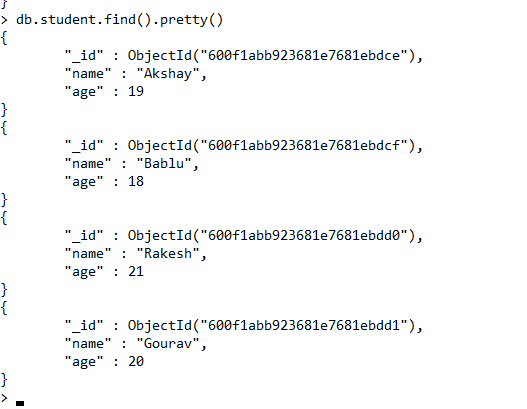• Return all the documents in ascending order of the age:
`db.student.find().sort({age:1})`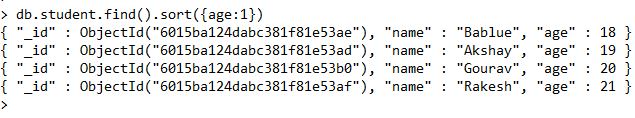• Return all the documents in descending order of the age:
`db.student.find().sort({age:-1})`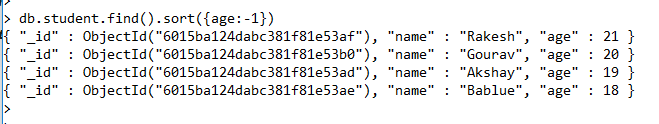• Return all the documents in the ascending order of the age:
`db.student.find().sort({name:1})`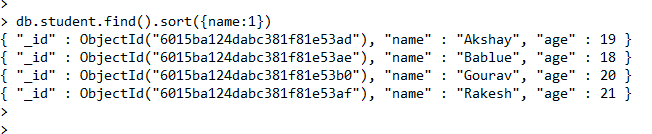• Return all the documents in the descending order of the age:
`db.student.find().sort({name:-1})`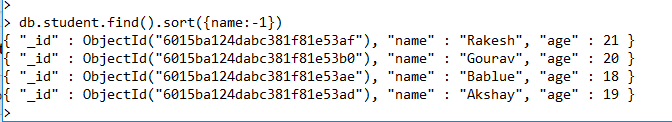My Personal Notes arrow_drop_up